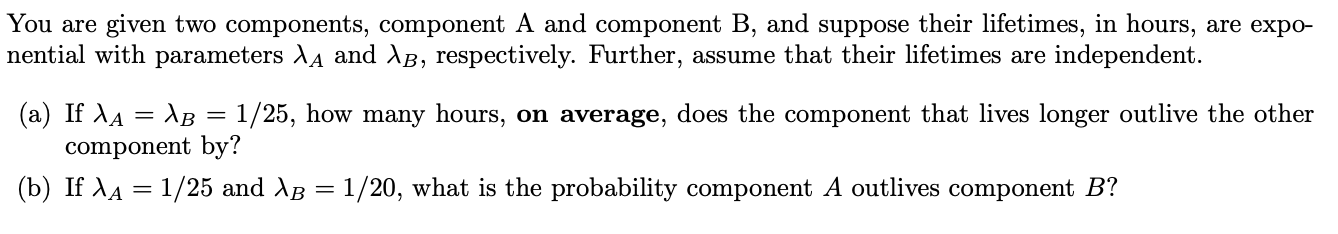# You are given two components, component A and component B, and suppose their lifetimes, in hours, are expo-nential with parameters XA and AB, respectively. Further, assume that their lifetimes are independent.(a) If AA AB = 1/25, how many hours, on average, does the component that lives longer outlive the othercomponent by?(b) If AA 1/25 and AB = 1/20, what is the probability component A outlives component B?

Question
1 viewshelp_outlineImage TranscriptioncloseYou are given two components, component A and component B, and suppose their lifetimes, in hours, are expo- nential with parameters XA and AB, respectively. Further, assume that their lifetimes are independent. (a) If AA AB = 1/25, how many hours, on average, does the component that lives longer outlive the other component by? (b) If AA 1/25 and AB = 1/20, what is the probability component A outlives component B? fullscreen
check_circle

Step 1

According to the provided data,

Step 2

a. The concept of memory less property is used here, that is, if X~exp(λ), then P(X>t+s|X>s) = e-dt. Therefore, even elapsing or expanding ‘s’ unit of time X still follows exponen...

### Want to see the full answer?

See Solution

#### Want to see this answer and more?

Solutions are written by subject experts who are available 24/7. Questions are typically answered within 1 hour.*

See Solution
*Response times may vary by subject and question.
Tagged in
MathProbability

### Random Processes